Subsections

## September 23

1.
Draw a plot of the single particle distribution function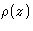as a function of z for the liquid monolayer, adsorbed on the flat surface. z is the distance perpendicular to the adsorbing surface. Consider a liquid of hard-spheres of diameter d.

Solution:
There are molecules at the distance d/2 from the wall only (we have a monolayer), sohas a sharp peak: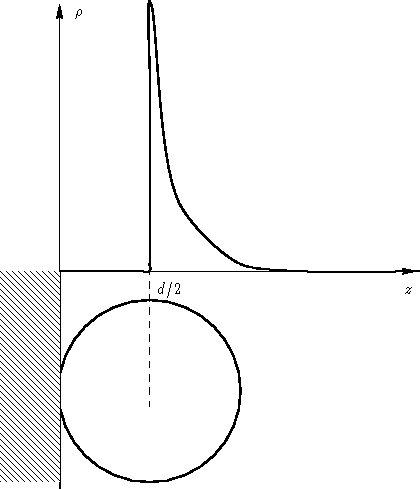2.
How is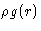normalized?
Solution:
The expression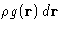is the average number of particles in the volume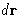around the point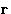if one particle is in the point. Integrating this, we obtain N-1 (one particle is at the point!).
3.
Doesat large distances in the perfect crystal?
Solution:
The function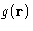does not. Crystal has long range order: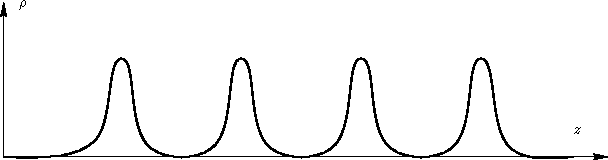When we average integrate over, we obtain many peaks, but they do not tend to 1.

4.
Is the derivation of the equations (3-9) valid for the anisotropic liquid crystals, or for crystalline solids?
Solution:
As long as we don't introduce spherical symmetry and integrate over dr, our equations are valid

## September 30

1.
Equipartition theorem works
(a)
For high temperatures
(b)
For low temperatures
(c)
Always
(d)
Never
Solution:
Since classical limit is for high temperatures (difference between levels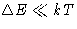), the answer (1a) is right

2.
Consider a cubic crystal with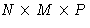atoms, N<M<P. The minimal value of q is
(a)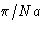(b)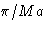(c)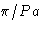(d)(e)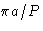(f)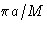(g)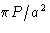(h)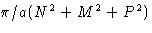Solution:
The values for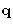are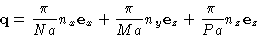where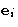is the unit vector along the corresponding axis. ThenThis is minimal at nx=ny=0, nz=1. The correct answer is (2c).

## October 2

1.
Sketch the phase diagram for two phase equilibrium in P-T and V-T coordinates.
Solution:
Since P is an intensive parameter, and V is an extensive one, we have: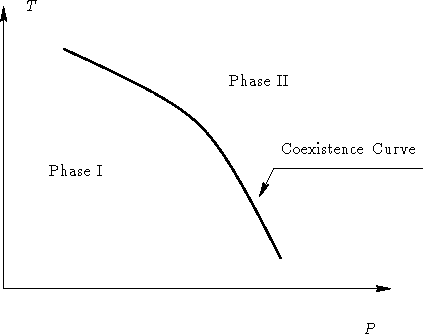and in V-T space: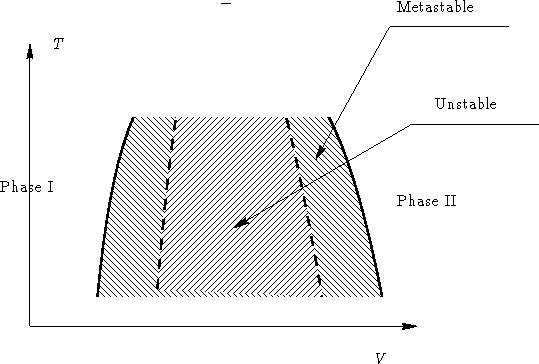2.
Same near triple point.
Solution:
See picture: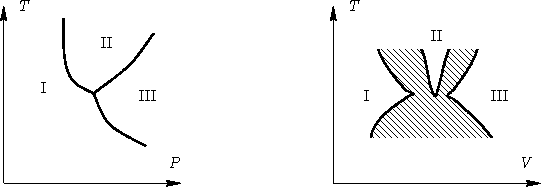3.
Consider a one-component system in an external electric field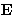. It can have dipole moment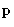parallel to. How many phases can coexist in this system?
Solution:
We add a scalar parameter |E|. Therefore the number of phases is 3+1=4.
4.
Same for binary blend in electric field.
Solution:
5 (see above)Next: Phase Transitions Up: Thermodynamics of Phase Transitions Previous: Thermodynamics of Phase Transitions

© 1997 Boris Veytsman and Michael Kotelyanskii
Tue Oct 7 22:16:36 EDT 1997